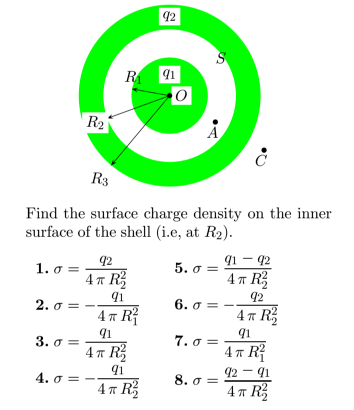# Problem: A solid conducting sphere of radius R1 and total charge q1 is enclosed by a conducting shell with an inner radius R2 and outer radius R3 and total charge q2. OA = a and OC = c.

99% (7 ratings)
###### Problem Details

A solid conducting sphere of radius R1 and total charge q1 is enclosed by a conducting shell with an inner radius R2 and outer radius R3 and total charge q2OA = a and OC = c.Frequently Asked Questions

What scientific concept do you need to know in order to solve this problem?

Our tutors have indicated that to solve this problem you will need to apply the Gauss' Law concept. You can view video lessons to learn Gauss' Law. Or if you need more Gauss' Law practice, you can also practice Gauss' Law practice problems.

How long does this problem take to solve?

Our expert Physics tutor, Juan took 2 minutes and 28 seconds to solve this problem. You can follow their steps in the video explanation above.

What professor is this problem relevant for?

Based on our data, we think this problem is relevant for Professor Perry's class at TEXAS.